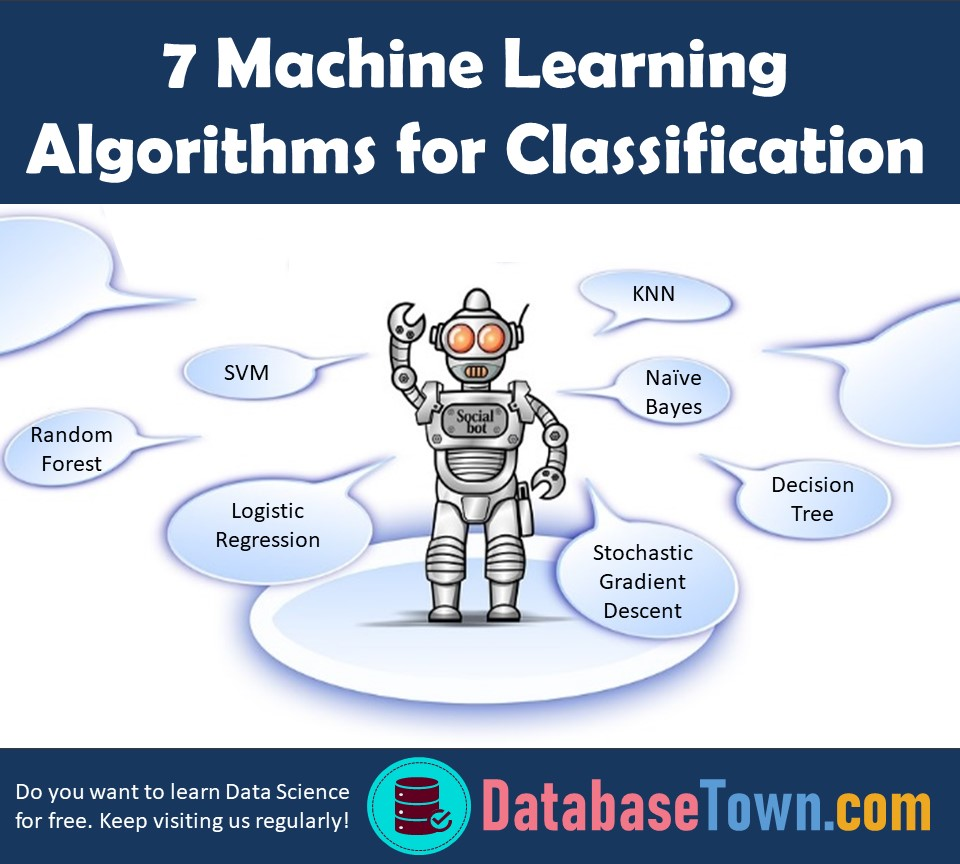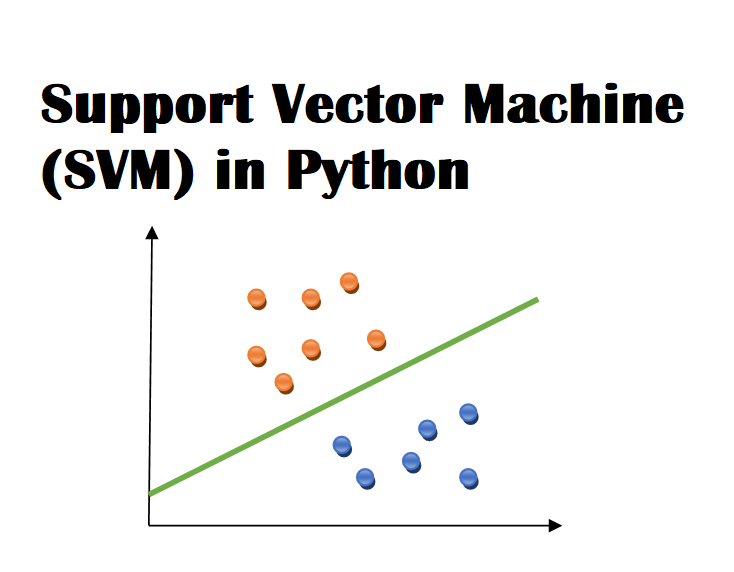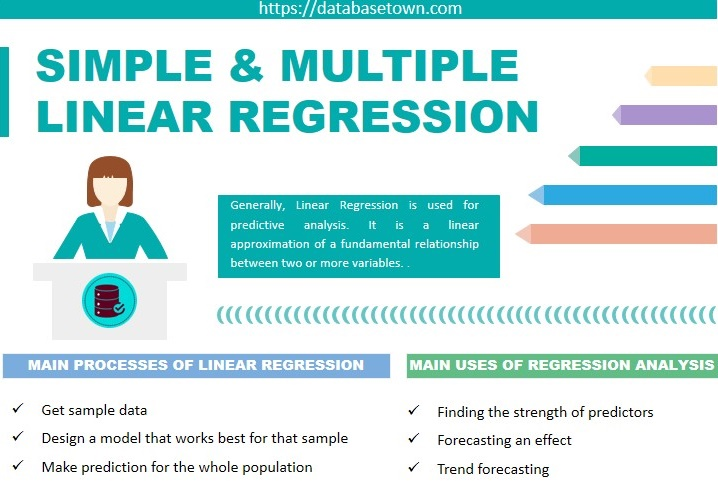# Machine Learning Algorithms## 7 Commonly Used Machine Learning Algorithms for Classification

Generally, data is a set of factual information based on numbers, words, observations, measurements that can be used for calculation, discussion and reasoning. The rough dataset is the essential foundation of data science and it may be of diverse types, such as structured data and unstructured data and semi-structured. Structured data is formatted in tabular …## Implementing Support Vector Machine (SVM) in Python

Machine Learning is the most famous procedure of foreseeing the future or arranging data to help individuals in settling on essential choices. The algorithms are trained over models through which they gain information from past encounters so as to make forecasts about what’s to come. There are three types of Machine learning i.e. supervised learning, …## What is Clustering & its Types? K-Means Clustering Example (Python)

Cluster Analysis Cluster is a group of data objects that are similar to one another within the same cluster, whereas, dissimilar to the objects in the other clusters. Cluster analysis is a technique used to classify the data objects into relative groups called clusters. Clustering is an unsupervised learning approach in which there are no …## Logistic Regression (Python) Explained using Practical Example

Logistic Regression is a predictive analysis which is used to explain the data and relationship between one dependent binary variable and one or more nominal, ordinal, interval or ratio-level independent variables. It is mostly used in biological sciences and social science applications. For instance, predict whether received email is spam or not. Similarly, predict whether …## Simple and Multiple Linear Regression in Python

Generally, Linear Regression is used for predictive analysis. It is a linear approximation of a fundamental relationship between two or more variables. Main processes of linear regression Get sample data Design a model that works best for that sample Make prediction for the whole population Main uses of regression analysis Finding the strength of predictors …